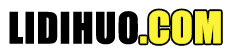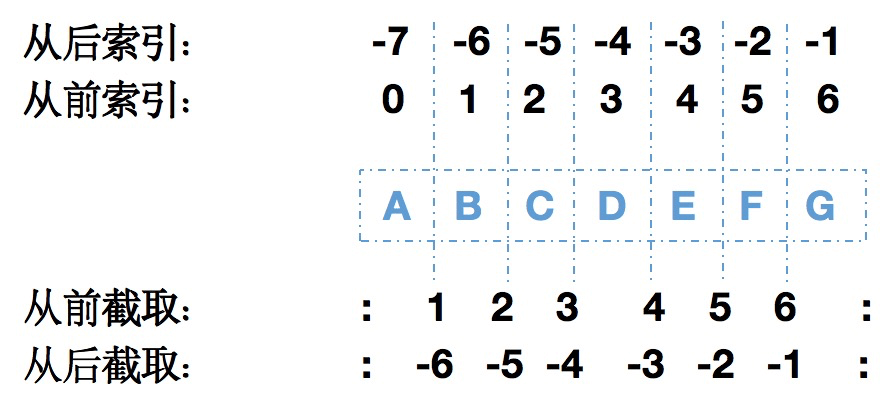# Python 数据类型

## 文本类型

### 1、对引号进行转义

str = 'I\'m a lidihuo'
print(str)

I'm a lidihuo

### 2、不同的引号

str1 = "I'm a lidihuo"
str2 = 'Hello " World'
print(str1)
print(str2)

I'm a lidihuo
Hello " World

### 3、长字符串换行

Python中对程序的换行和缩进都有严格的语法要求。当一个较长字符串需要换行时需要在行尾添加反斜杠 \ ，否则会出现语法报错，比如：
str = 'In programming, data type is an important concept,\
Variables can store data of different types, and different types can do different things.'

str2 = 'Hello " World'
print(str)

In programming, data type is an important concept,Variables can store data of different types, and different types can do different things.

### 4、字符串截取

python中字符串截取索引值0 为从头开始，-1 为从末尾的开始。str = 'helloworld'
print(str) # 输出字符串
print(str[0:-1]) # 输出第1个到倒数第2个之间的字符
print(str) # 输出字符串第1个字符
print(str[2:5]) # 输出从第3个开始到第5个的字符
print(str[2:]) # 输出从第3个开始的后的所有字符
print(str * 2) # 输出字符串2次，也可以写成 print (2 * str)
print(str + "I am lidehuo") # 连接字符串

helloworld
helloworl
h
llo
lloworld
helloworldhelloworld
helloworldI am lidehuo

## 数值类型

a = 10
b = 0
c = -100
d = 1_234_567
print(a)
print(b)
print(c)
print(d)

10
0
-100
1234567

### 2、float (浮点数)

a = 12.34
b = 100000000000000000000.45
print("a = ", a)
print("b = ", b)

a = 12.34
b = 1e+20

### 3、complex（复数）

complex是 Python 的内置类型，由实数部分和虚数部分构成，虚数部部用j或者J作为后缀，比如：
a = 1.2 + 1.2j
print(a = ", c1)
print("a type is ", type(c1))

a = (1.2+1.2j)
a type is

## 序列类型

### 1、list（列表）

list（列表）是 Python 中最常用的数据类型，list中的元素可以不同，支持数字、字符串和嵌套列表本身。
list的元素在方括号 [] 之间，并用逗号进行分隔，格式为：[元素,元素]。
list的索引值以 0 为开始值，-1 为从末尾的开始位置。
list可以根据索引进行截取，被截取后仍然是一个列表。list（列表）具体操作如下：
list1 = ['a','b','c','d','e']
list2 = [123,'abcd']
print(list1) # 输出完整列表
print(list1) # 输出列表第1个元素
print(list1[1:3]) # 从第2个开始输出到第3个元素
print(list1[2:]) # 输出从第3个元素开始的所有元素
print(list2*2) # 输出2次列表
print(list1+list2) # 连接列表

['a', 'b', 'c', 'd', 'e']
a
['b', 'c']
['c', 'd', 'e']
[123, 'abcd', 123, 'abcd']
['a', 'b', 'c', 'd', 'e', 123, 'abcd']

a = ['a','b','c','d','e']
a = 'f'
a[2:4] = ['a','b','c','d','e']
print(a)
a[2:4] = []
print(a)

['f', 'b', 1, 2, 'e']
['f', 'b', 'e']

### 2、tuple(元组)

tuple与列表类似，不同之处在于tuple的元素不能修改。tuple写在小括号 () 里，元素之间用逗号隔开，tuple中的元素类型可以不同，具体操作如下：
tuple1 = ('a','b','c','d','e')
tuple2 = (123,'abcd')
print(tuple1) # 输出完整元组
print(tuple1) # 输出元组第1个元素
print(tuple1[1:3]) # 从第2个开始输出到第3个元素
print(tuple1[2:]) # 输出从第3个元素开始的所有元素
print(tuple2*2) # 输出2次元组
print(tuple1+tuple2) # 连接元组

['f', 'b', 1, 2, 'e']
['f', 'b', 'e']
('a', 'b', 'c', 'd', 'e')
a
('b', 'c')
('c', 'd', 'e')
(123, 'abcd', 123, 'abcd')
('a', 'b', 'c', 'd', 'e', 123, 'abcd')

### 3、range (范围)

range 类似于list，是范围列表，不过里面的元素只能是数字，在for循环中应用较多。具体格式range(start, stop, step)
start: 计数从 start 开始。默认是从 0 开始。 stop: 计数到 stop 结束，但不包括 stop。 step：步长，默认为1。
range(10) # 等价于range(0,10)
range(5,10) #5,6,7,8,9,10
range(10,100,10) # 10，20，30...

for i in range(5):
print(i)

0
1
2
3
4
for i in range(0,6,2):
print(i)

0
2
4
for i in range(5,0,-1):
print(i)

5
4
3
2
1
l = [1, "range", "的", "for", "循环"]
for i in range(len(l)):
print(i)

0
1
2
3
4

## 映射类型

### dict（字典）

dict是无序可变的序列，元素以“键值对（key-value）”的形式存储。 dict相对于列表（list）和元组（tuple）是有序的序列。 dict中的元素是通过键来存取的，列表是通过下标索引存取的。 dict用 { } 标识，是一个键(key) : 值(value) 的集合，key值必须保障唯一。 dict格式为{'key':'value1', 'key2':'value2', ..., 'keyn':valuen}

dict = {}
dict['name'] = "lidihuo"
dict = "Hello World"
dict1 = {'name': 'lidihuo','course':'python', 'site': 'www.lidihuo.com'}
dict2 = {(1,2,3): 'great', 30: [1,2,3]} # 使用元组和列表作为key和value
print(dict['name']) # 输出键为 'name' 的值
print(dict) # 输出键为1的值
print(dict1) # 输出完整的字典
print(dict1.keys()) # 输出所有键
print(dict1.values()) # 输出所有值
print(dict2) # 输出完整的字典

lidihuo
Hello World
{'name': 'lidihuo', 'course': 'python', 'site': 'www.lidihuo.com'}
dict_keys(['name', 'course', 'site'])
dict_values(['lidihuo', 'python', 'www.lidihuo.com'])
{(1, 2, 3): 'great', 30: [1, 2, 3]}

dict是通过键来访问对应的值。因为字典中的元素是无序的，每个元素的位置都不固定，所以字典也不能像列表和元组那样，采用下标来访问元素。
dict元素的具体格式为：dictname[key]。dictname 表示dict变量的名字，key 表示键名。
dict = {'name': 'lidihuo','course':'python', 'site': 'www.lidihuo.com'}
print(dict['name']) # 输出键对应的值

lidihuo
Python 中更推荐使用get() 方法来获取指定键对应的值。语法格式为：dictname.get(key[,default])，其中，dictname 表示字典变量的名字；key 表示指定的键； default 用于指定要查询的键不存在时，此方法返回的默认值，如果不手动指定，会返回 None。
dict = {'name': 'lidihuo','course':'python', 'site': 'www.lidihuo.com'}
print(dict.get('name'))

lidihuo

## 集合类型

### 1、set集合

a = {1,'a',(1,2,3),'b',1.2} 用{}方式创建
b = set((1,2,3,4,5)) set方式创建
print(a)
print(b)

{1, 1.2, (1, 2, 3), 'b', 'a'}
{1, 2, 3, 4, 5}

a = {'cat', 'dog', 'cow', 'pig'}
print(a)
for item in a:
print(item)

{'cow', 'cat', 'pig', 'dog'}
cow
cat
pig
dog
set集合之间运算
a = set('123456789')
b = set('45678')
print(a)
print(a - b) # a 和 b 的差集
print(a | b) # a 和 b 的并集
print(a & b) # a 和 b 的交集
print(a ^ b) # a 和 b 中不同时存在的元素

{'7', '9', '3', '6', '1', '8', '5', '2', '4'}
{'2', '9', '3', '1'}
{'7', '9', '3', '6', '1', '8', '5', '2', '4'}
{'7', '6', '8', '5', '4'}
{'9', '3', '1', '2'}

### frozenset 集合

frozenset 和set相比是不可变序列，所有在set中能改变集合本身的方法，比如 remove()、discard()、add() 等，frozenset 都不支持.

a = {'cat', 'dog', 'cow', 'pig'}
b = frozenset(['lion', 'deer'])
c = {'rabbit', 'rat'}
# 向set集合中添加frozenset
a.add(b)
print(b)
# 向为set集合添加子set集合
c.add(a)
print(c)
print(a | b) # a 和 b 的并集
print(a & b) # a 和 b 的交集
print(a ^ b) # a 和 b 中不同时存在的元素

{'cow', frozenset({'deer', 'lion'}), 'dog', 'pig', 'cat'}
Traceback (most recent call last):
File "script.py", line 8, in
c.add(a)
TypeError: unhashable type: 'set'
Exited with error status 1

## 布尔类型

>>> True or True
True
>>> True and False
False
>>> not Flase
True
if 1>2:
print('1>2')
elif1==2:
print('1>2')
else:
print('1<2')

1<2

## 8、二进制类型

### bytes（字节串）

bytes是 Python 3.x 新增的类型，字符串由若干个字符组成，以字符为单位进行操作 字节串和字符串除了操作的数据单元不同之外，它们支持的所有方法都基本相同。 字节串和字符串都是不可变序列，不能随意增加和删除数据 bytes 以字节序列的形式（二进制形式）来存储数据，比如字符串、数字、图片、音频等。
a = bytes()
b = b'lidihuo'
c = bytes('立地货', encoding='UTF-8')
d = "立地货".encode('UTF-8')
print(a)
print(b)
print(c)
print(d)

b''
b'lidihuo'
b'\xe7\xab\x8b\xe5\x9c\xb0\xe8\xb4\xa7'
b'\xe7\xab\x8b\xe5\x9c\xb0\xe8\xb4\xa7'

## 制定数据类型

 操作 数据类型 x = str("Hello World") str x = int(20) int x = float(20.5) float x = complex(1j) complex x = list(("apple", "banana", "cherry")) list x = tuple(("apple", "banana", "cherry")) tupe x = range(6) range x = dict(name="John", age=36) dict x = set(("apple", "banana", "cherry")) set x = frozenset(("apple", "banana", "cherry")) frozenset x = bool(5) bool x = bytes(5) bytes x = bytearray(5) bytearray x = memoryview(bytes(5)) memoryview
x = 1# int
y = 1.2# float
z = 1j# complex
a = float(x)# 转换称float
b = int(y)# 转换int
c = complex(x)# 转换称complex:
print(a)
print(b)
print(c)

1.0
1
(1+0j)
"内容整理不易，点下广告也是鼓励～"

Python有两种类型的类型转换：隐式类型转换、显式类型转换。

1、隐式类型转换

```num_int = 123
num_flo = 1.23
num_new = num_int + num_flo
print("datatype of num_int:",type(num_int))
print("datatype of num_flo:",type(num_flo))
print("Value of num_new:",num_new)
print("datatype of num_new:",type(num_new))```

2、显式类型转换

```num_int = 123
num_str = "456"
print("Data type of num_int:",type(num_int))
print("Data type of num_str before Type Casting:",type(num_str))
num_str = int(num_str)
print("Data type of num_str after Type Casting:",type(num_str))
num_sum = num_int + num_str
print("Sum of num_int and num_str:",num_sum)
print("Data type of the sum:",type(num_sum))```

Python中的每个值都有一个数据类型。由于在Python编程中一切都是对象，因此数据类型实际上是类，而变量是这些类的实例（对象）。

Python中有多种数据类型，具体如下：

1、文字类型： str，比如“Hello World”。

2、数值类型： int ，float，complex，比如：1、1.2、1.2+1.3j。

3、序列类型： list，tuple， range。比如：[a,b,c]、(a,b,c)。

4、映射类型： dict，比如：{“a”:1,”b”:2}。

5、集合类型： set，frozenset，比如：{‘a’,’b’}。

6、布尔类型： bool，比如，True、False。

7、二进制类型： bytes，bytearray， memoryview。

Copyright © 2020 立地货 All Rights Reserved.